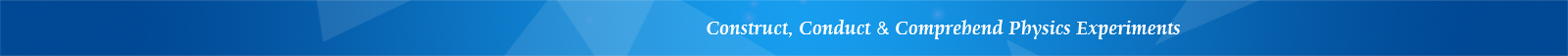## LEMI-16 Simple Pendulum with Electric Timer

Features

• Hall sensor and electronic timer

• Accurate angle and length measurements

• High measurement accuracy

Introduction

Pendulum experiment is important in general physics teaching. In the past, this experiment was limited to a small-angle approximation. Traditional methods using manual stopwatch timing have significant measurement errors, and multiple-period measurement and averaging method is used to reduce the measurement error. Due to the presence of air damping, swing angle gradually decays with time degrading the measurement accuracy.

This apparatus uses an integrated Hall sensor and electronic timer, which can accurately measure the period under large swing angle in a few swinging cycles, so the effect of air damping on pendulum angle can be ignored. Based on the relationship between the period and the angle, it is possible to acquire a precise value of the gravitational acceleration by extrapolating the angle to zero degree.

Using this apparatus, the following experiments can be conducted:

1. Measure the swinging period with a fixed string length, and calculate the gravitational acceleration.

2. Measure the swinging period by varying string length, and calculate the corresponding gravitational acceleration.

3. Verify the pendulum period is proportional to the square of the string length.

4. Measure the swinging period by varying initial swing angle, and calculate the gravitational acceleration.

5. Use extrapolation method to acquire accurate gravitational acceleration at extra small swinging angle.

6. Study the influence of non-linear effect under large swing angles.

Through these experiments, students will acquire the necessary knowledge, learn the basic experimental ideas, and understand the relationship among the wire length, the starting angle, and the gravitational acceleration.